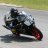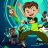# Fibonacci Fans

C

#### chewie76

##### New member
Last edited:#### BenTen

Staff
VIP
Warehouse
Give this a try

Rich (BB code):
``````#hint: <b>Fibonacci Fan lines</b>
Fibonacci Fan lines are trend lines based on Fibonacci retracement points. Rising fan lines extend up from a trough and pass through retracement based on the advance (lowest low to highest high or on the falling fan lines: highest high to lowest low). These fan lines can then be used to estimate support levels or potential reversal zones. Falling fan lines can then be used to estimate resistance levels or potential reversal zones.

#hint Price: Price used in the alerts on crossing of the fans. <b>(Default is Close)</b>

#hint onExpansion: Determines if the fan lines are projected past the current bar into the right side expansion <b>(Default is Yes)</b>

#hint Coefficient1: Fan Line 1: Trough to 38.2% retracement on rising fans.
Peak to 38.2% Coefficient on falling fans. <b>(Default is 38.2%)</b>

#hint Coefficient_2: Fan Line 2:
Trough to 50% retracement on rising fans.
Peak to 50% Coefficient on falling fans. <b>(Default is 50%)</b>

#hint Coefficient_3: Fan Line 3:
Trough to 61.8% retracement on rising fans.
Peak to 61.8% Coefficient on falling fans. <b>(Default is 61.8%)</b>

#wizard input: Price

#wizard text: Inputs: Price:

#wizard input: onExpansion

#wizard text: onExpansion:

#wizard input: Coefficient1

#wizard text: Coefficient1 :

#wizard input: Coefficient_2

#wizard text: Coefficient_2:

#wizard input: Coefficient_3

#wizard text: Coefficient_3:

input price = close;

input high = high;

input low = low;

input onExpansion = Yes;

input Coefficient1 = .382;

input Coefficient_2 = .5;

input Coefficient_3 = .618;

def a = HighestAll(high);

def b = LowestAll(low);

def barnumber = barNumber();

def c = if high == a then barnumber else double.nan;

def d = if low == b then barnumber else double.nan;

rec highnumber = compoundValue(1, if IsNaN(c) then highnumber else c, c);

def highnumberall = HighestAll(highnumber);

rec lownumber = compoundValue(1, if IsNaN(d) then lownumber else d, d);

def lownumberall = LowestAll(lownumber);

def upward = highnumberall > lownumberall;

def downward = highnumberall < lownumberall;

def low382 =  b + ((b - a) * Coefficient1);

def low5 =  b + ((b - a) * Coefficient_2);

def low618 = b + ((b - a) * Coefficient_3);

def high382 =  a - ((a - b) * Coefficient1);

def high5 =  a - ((a - b) * Coefficient_2);

def high618 = a - ((a - b) * Coefficient_3);

def x = AbsValue(lownumberall - highnumberall );

def slope = (a - b) / x;

def slope382 = (high382 - b) / x;

def slope5 = (high5 - b) / x;

def slope618 = (high618 - b) / x;

def slopelow = (b - a) / x;

def slopelow382 = (low382 - b) / x;

def slopelow5 = (low5 - b) / x;

def slopelow618 = (low618 - b) / x;

def day = getDay();

def month = getMonth();

def year = getYear();

def lastDay = getLastDay();

def lastmonth = getLastMonth();

def lastyear = getLastYear();

def isToday = if(day == lastDay and month == lastmonth and year == lastyear, 1, 0);

def istodaybarnumber = HighestAll(if isToday then barnumber else double.nan);

def line = b + (slope * (barnumber - lownumber));

def line382 = b + (slope382 * (barnumber - lownumber));

def line5 = b + (slope5 * (barnumber - lownumber));

def line618 = b + (slope618 * (barnumber - lownumber));

def linelow = a + (slopelow * (barnumber - highnumber));

def line382low = a + (slopelow382 * (barnumber - highnumber));

def line5low = a + (slopelow5 * (barnumber - highnumber));

def line618low = a + (slopelow618 * (barnumber - highnumber));

def currentlinelow = if barnumber <= istodaybarnumber then linelow else double.nan;

def currentline382low = if barnumber <= istodaybarnumber then line382low else double.nan;

def currentline5low = if barnumber <= istodaybarnumber then line5low else double.nan;

def currentline618low = if barnumber <= istodaybarnumber then line618low else double.nan;

def currentline = if barnumber <= istodaybarnumber then line else double.nan;

def currentline382 = if barnumber <= istodaybarnumber then line382 else double.nan;

def currentline5 = if barnumber <= istodaybarnumber then line5 else double.nan;

def currentline618 = if barnumber <= istodaybarnumber then line618 else double.nan;

Plot FibFan =  if  downward and onExpansion then linelow else if downward then currentlinelow else if upward and onExpansion then line else if upward then currentline else double.nan;

FibFan.SetStyle(Curve.SHORT_DASH);

FibFan.AssignValueColor(color.red);

Plot "Coefficient 1" =  if (downward and onExpansion) then line382low else if downward then currentline382low else if upward and onExpansion then line382 else if upward then currentline382 else double.nan;

"Coefficient 1".SetStyle(Curve.Firm);

"Coefficient 1".AssignValueColor(color.red);

Plot "Coefficient 2" = if downward and onExpansion then line5low else if downward then currentline5low else if upward and onExpansion then line5 else if upward then currentline5 else double.nan;

"Coefficient 2".AssignValueColor(color.red);

"Coefficient 2".SetStyle(Curve.Firm);

Plot "Coefficient 3" =  if downward and onExpansion then line618low else if downward then currentline618low else if upward and onExpansion then line618 else if upward then currentline618  else double.nan;

"Coefficient 3".AssignValueColor(color.red);

"Coefficient 3".SetStyle(Curve.Firm);

alert((price crosses below "Coefficient 1") , "Price crosses below Coefficient 1");

alert((price crosses below "Coefficient 2") , "Price crosses below Coefficient 2");

alert((price crosses below "Coefficient 3") , "Price crosses below Coefficient 3");

alert((price crosses above "Coefficient 1") , "Price crosses above Coefficient 1");

alert((price crosses above "Coefficient 2") , "Price crosses above Coefficient 2");

alert((price crosses above "Coefficient 3") , "Price crosses above Coefficient 3");

End``````

Last edited:#### BenTenCustom 0Custom 16Custom 11